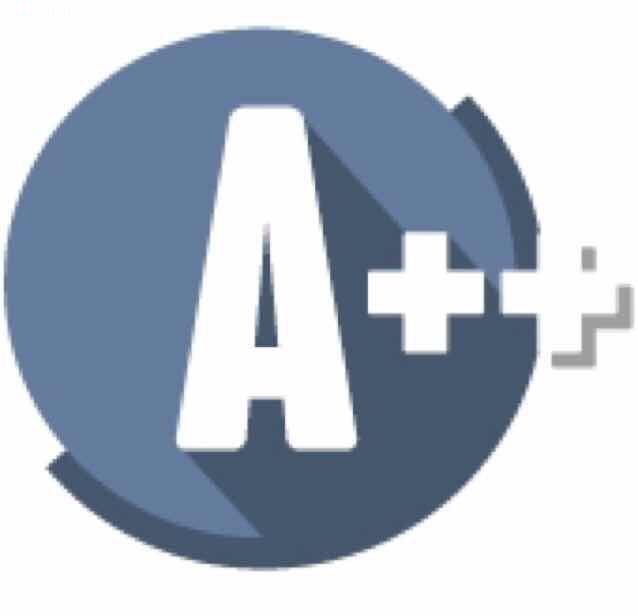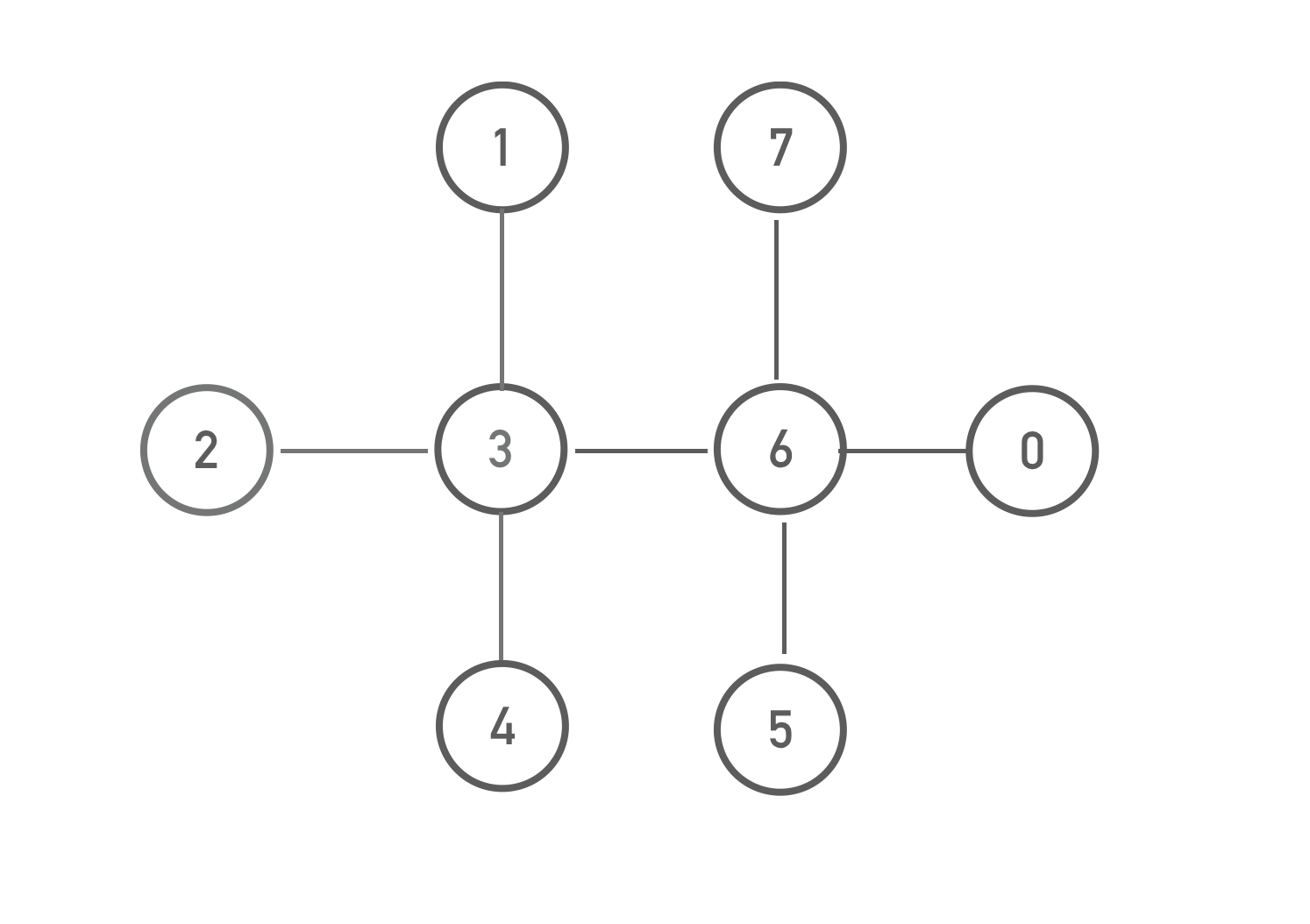## Interviews

#### Find Critical NodesGiven an undirected graph, find out all the vertices when removed will make the graph disconnected.  Initially the graph is connected.

For example given the graph below:Return [3, 6]. Because we can make the graph disconnected by removing either vertex 3 or vertex 6.

Input:

nodeNum, the total count of vertices in the graph. Each vertex has an unique id in the range from 0 to nodeNum - 1 inclusive.

edges, a list of integer pairs representing all the edges on the graph.

output:

A list of integers representing the ids of the articulation points.

example:

Input:

nodeNum = 7

edges = [[0,1], [0, 2], [1, 3], [2, 3], [2, 5], [5, 6], [3,4]]

Output:

[2,3,5]

##### Solve the problem:

def findCriticalNodes(nodeNum, edges):